# Texas Go Math Grade 1 Lesson 2.1 Answer Key Greater Than

Refer to our Texas Go Math Grade 1 Answer Key Pdf to score good marks in the exams. Test yourself by practicing the problems from Texas Go Math Grade 1 Lesson 2.1 Answer Key Greater Than.

## Texas Go Math Grade 1 Lesson 2.1 Answer Key Greater Than

Essential Question
How can you compare two numbers to find which is greater?
Explanation:
First we will check the tens then ones
When the digits in hundreds place are equal, then the number with more number of tens is a greater number. 4. When the digits in tens place are equal, then the number with more number of ones is a greater number.

Explore
Useto solve.
Draw quick pictures to show your work.For The Teacher

• Read the problem. Which number is greater, 65 or 56? Have children use base-ten blocks and draw quick pictures to solve.Explanation:
1 ten = 10 ones
6 tens = 60 and 5 ones = 65
5 tens = 50 and 6 ones = 56
so, 65 > 56.
6 tens are greater than 5 tens.

Math Talk
Mathematical Processes
How did you decide which number is greater? Explain.
Explanation:
First we will check the tens then ones
When the digits in hundreds place are equal, then the number with more number of tens is a greater number. 4. When the digits in tens place are equal, then the number with more number of ones is a greater number.

Model and Draw
To compare 25 and 17, first compare the tens.Explanation:
First we will check the tens then if tens are equal then we have to check the ones

If the tens are the same, compare the ones.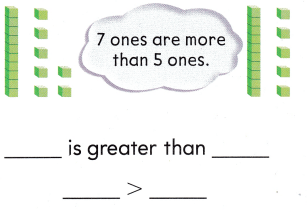Explanation:
If tens place are same then we compare the ones

Share and Show

Use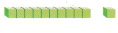to show each number.

Question 1.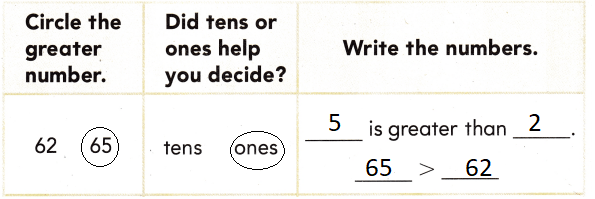Explanation:Question 2.Explanation:Question 3.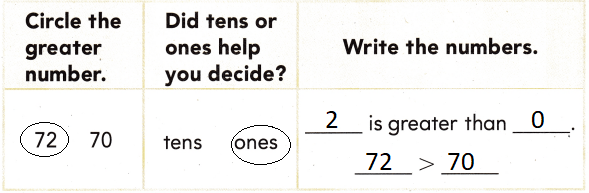Explanation:Problem Solving

Useif you need to.

Question 4.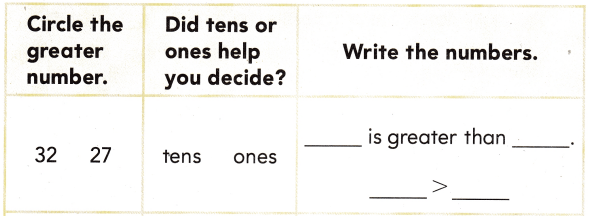Explanation: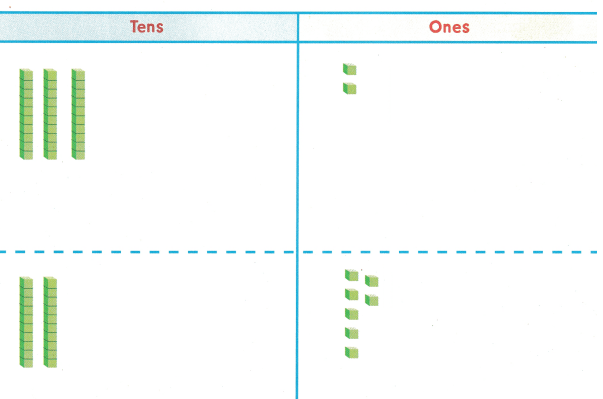Question 5.Explanation: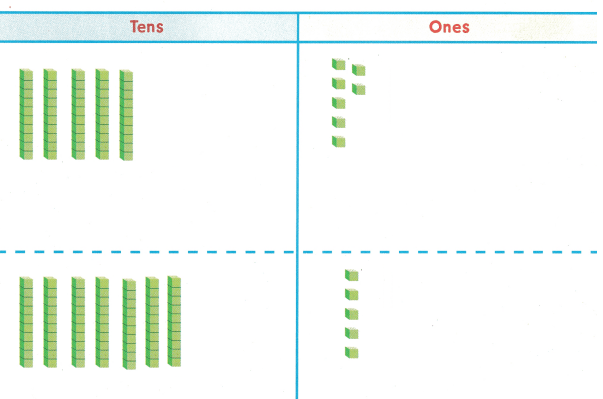Write or draw to solve.

Question 6.
H.O.T. Pam and Jake play a game for points. Pam’s points are 1 ten 6 ones. Jake’s points are 1 one 6 tens. Who has the greater number of points?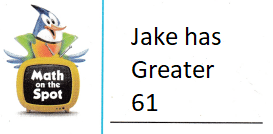Explanation:
Pam’s points are 1 ten 6 ones. = 16
Jake’s points are 1 one 6 tens. = 61
61 > 16
6 is greater than 1

Question 7.
H.O.T. Multi-Step John has 51 cards. Paul has 32 cards. Ben has a stack of cards greater than either Paul or John. How many cards might Ben have?
__ cards
cards
Explanation:
John has 51 cards. Paul has 32 cards.
Ben has a stack of cards greater than either Paul or John.
That might be 52
52 > 51

Question 8.
You find 42 crackers. You look for more crackers. Which number is greater than 42?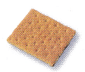(A) 42
(B) 51
(C) 38
Explanation:
Compared the tens
51 > 42
5 is greater than 4

Question 9.
You have 25 marbles. Which number is greater than 25?
(A) 22
(B) 50
(C) 21Explanation:
Compared the tens
50 > 25

Question 10.
Analyze Which number is greater than 63?
(A) 102
(B) 36
(C) 61
Explanation:
Compared the tens
102 > 63

Question 11.
Texas Test Prep Which number is greater than 67?
(A) 58
(B) 15
(C) 76
Explanation:
76 > 67
Compared the tens
7 > 6

Take Home Activity

• Write 38, 63, 68, and 83 on slips of paper. Show your child two numbers, and ask which number is greater. Repeat with different pairs of numbers.
Explanation:
38 and 63
63 > 38
63 and 68
68 > 63
38 and 83
83 > 38

### Texas Go Math Grade 1 Lesson 2.1 Homework and Practice Answer Key

Use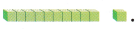Question 1.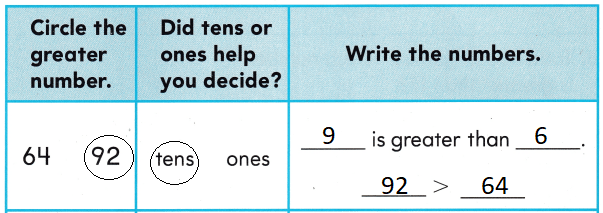Explanation:Question 2.Explanation:Question 3.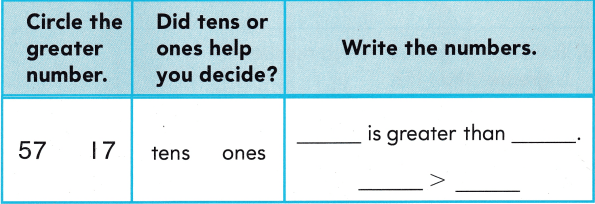Explanation: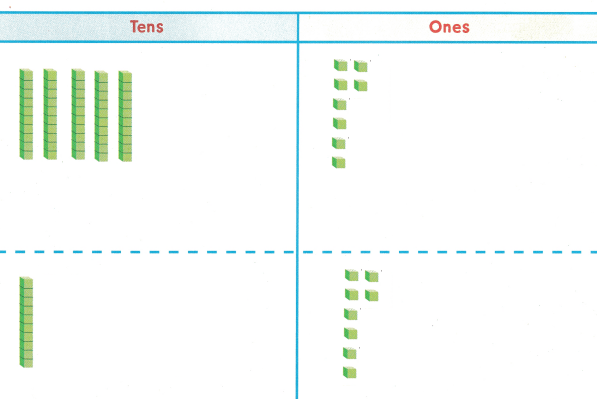Problem Solving

Question 4.
Jin counts 43 windows on the front of the school. He counts 47 windows on the back of the school. Which number of windows is greater than 45 windows?
__ windows
47 > 45 windows
Explanation:
If tens are same we have to compare the ones

Texas Test Prep

Question 5.
You count 53 pieces of popcorn. Which number is greater than 53?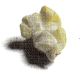(A) 61
(B) 50
(C) 49
Explanation:
If tens are same we have to compare the ones
61 > 53

Question 6.
Todd has 17 games. Which number is greater than 17?
(A) 16
(B) 10
(C) 30
Explanation:
If tens are same we have to compare the ones
30 > 17

Question 7.
Which number is greater than 87?
(A) 77
(B) 97
(C) 80
Explanation:
If tens are same we have to compare the ones
97 > 87

Question 8.
Which number is greater than 34?
(A) 52
(B) 29
(C) 33
Explanation:
52 > 34
If tens are same we have to compare the ones

Question 9.
Which number is greater than 47?
(A) 42
(B) 44
(C) 48